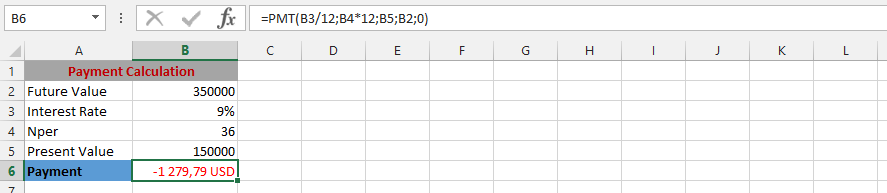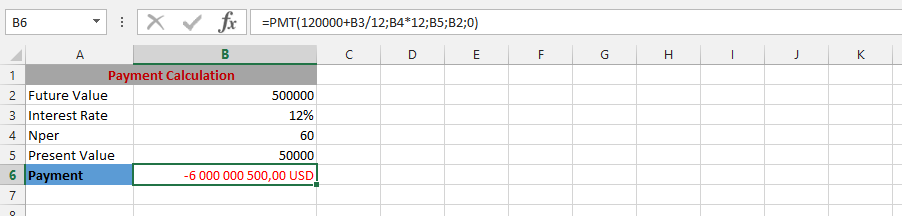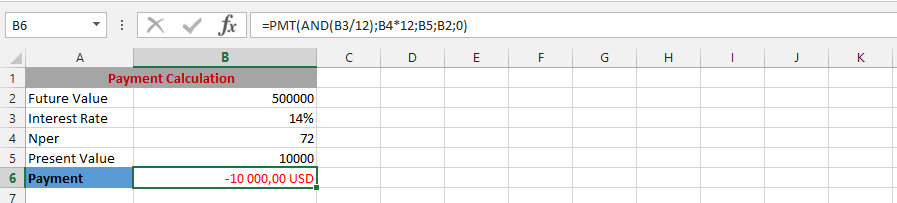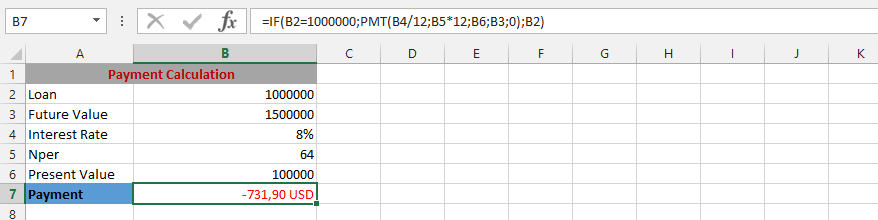### PMT Function In Excel and 13 Different Formulas

In this lesson you can learn how to calculate loan installment using PMT function in Excel.

PMT function helps you to calculate the amount of the installment loan assuming a fixed interest rate and fixed monthly payment amount.

In this feature, you can also calculate the amount you need to deposit to save a certain amount at a certain time.

Payments calculated by PMT contain basis and exclude taxes and interest, and other fees associated with the loan.

This function has the following syntax:

=PMT(rate,nper,pv,[fv],[type])

• Rate – the rate of interest loans.
• Nper is the total number of installments
• PV – current value (for example the amount of credit)
• FV – value or future financial level you want to get after the last payment
• Type is the number 0 or 1 indicates when the payment takes place 1-payment in advance, 0-payment of arrears.

### Example 1: Simple PMT Formula

We are evaluating a loan assessment, and want to know the payment, which is why we are using the PMT formula to find the answer.### Example 2: Complete PMT Formula

The client wants to acknowledge the payment, and what it would worth in the future. This in combine will allow the acknowledgement of the whole payment, with future value included.### Example 3: PMT Formula

The formula has been layout, and things are more complex. This is why we are using the formula to find the answer.### Example 4: Specific Amount Plus PMT Formula

The client has already layout a specific amount of money, which would be added, to acknowledge the whole payment. This is why we will be using the PMT formula for answers.### Example 5: PMT minus Percentage

The situation here is going to be crazy, because we do not know how it would work. We are considering increasing the percentage of the earnings. This also makes it necessary to find out the best way that work for the company. But, we already know the PMT, but want to know the increased amount of money.### Example 6: Average and PMT

The business is working very well, and we would like to acknowledge adding the average of the revenues to know how the performance work. This is why we will be using the AVERAGE to find how we will perform in the payment, and use it in combination to the PMT.### Example 7: Golden Client: SUM and PMT

It is our company’s Christmas gift, and we are just enjoying the Christmas gift. The company was given the opportunity to receive the company that is establishing different revenues, and this is why we are adding the all revenue, which they would like to pay back for 25 years. Now, we are using both SUM and PMT function to find out how much the opportunities would provide for 25 years to come.### Example 8: PMT & AND function

Our business is going well, and we would like to find out how the information would turn out how the business will go, and PMT & AND are being simultaneously used.### Example 9: PMT and SUM

The problem is quite different, because we do not know what to work, and the situation is now very different, and we need to use both the PMT and SUM for the successfulness, which would allow this to work out effectively.### Example 10: IF and PMT

A situation has come to light, and we need to acknowledge what it means to the firm. A client has informed us that if the loan is a specific amount, then he would like to extend the payment period for additional five years. We do not know the best method for dealing with it. But, we’d know that the situation can be resolved. The financial engineer was given the assignment to find the appropriate answer.### Example 11 Calculating monthly installment

Calculate the monthly installment of the loan in the amount of 100 000 \$. Interest is 7% per annum, and the loan must be paid within 15 months.

In any cell, enter the function:

=PMT (7%/12, 15, -100000)

• 7%/12 is interest rate per month
• number of rates is 15
• amount of credit is 100 000 (with minus because that’s not your money and you have to pay it back)

Result is 6 982\$

### Example 12 Calculating monthly installment paying in the beginning of the month

Calculate the monthly payment amount for the same loan, if payments with payment in advance (in the beginning of the month)

Formula is:

=PMT (7%/12, 15, -100000, 0, 1)

• 7%/12 is interest rate per month
• number of rates is 15
• amount of credit is 100 000 (with minus because that’s not your money and you have to pay it back)
• future value is 0, because you want to pay back whole loan
• 1 means payments in beginning of the period

Result is 6 941,51\$

### Example 13 How much to save to become millionaire?

You want to save \$ 1 000 000 over 10 years. Every year you deposit a fixed amount. The interest rate is 5% savings. Using the PMT function, calculate how much you should deposit per month.

Formula is:

=PMT (5%/12, 120, 0, 1000000)

• 5%/12 is interest rate per month
• 120 is number of month (10 years * 12)
• 0 is present value (you have any deposit now)
• 1 000 000\$ is future value

Result is -6 439,88\$ (with minus because you have to pay it to the bank)

## Template

```Further reading: